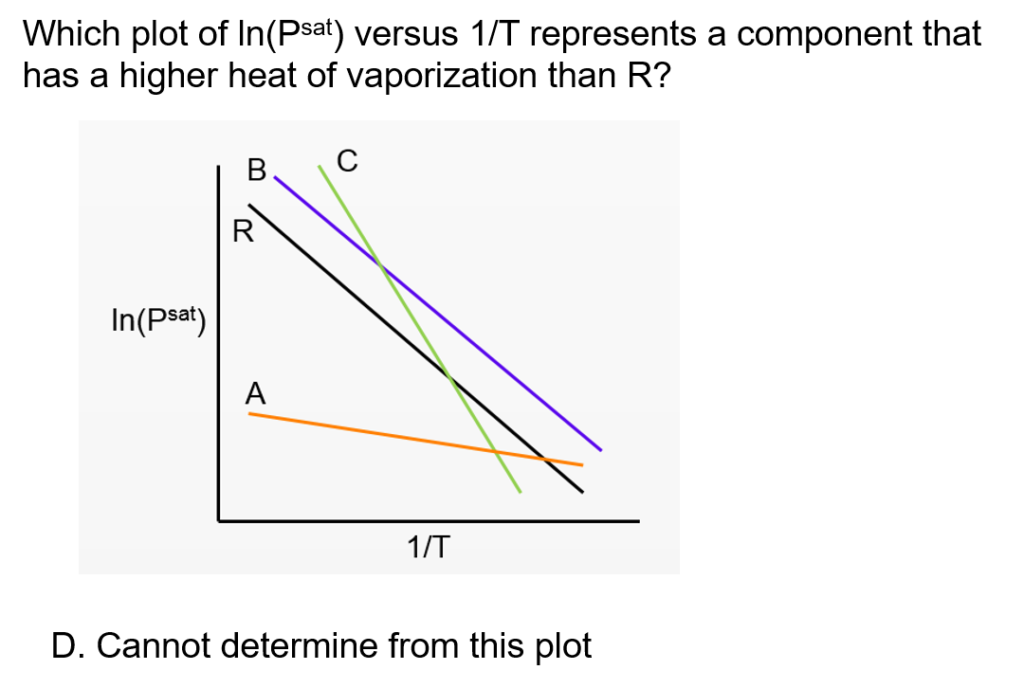#### Clapeyron, Clausius-Clapeyron, and Antoine Equations: ConcepTest and Example Problem

Try to answer this ConcepTest and solve the example problem before using this module. Studies show that trying to answer the questions before studying material improves learning and retention. We suggest that you write down the reasons for your answers. By the end of this module, you should be able to answer these on your own. Answers will be given at the end of this module.##### Example Problem 1

The heat of fusion of ice to form water is 335 J/g. The densities of water and ice are 1.00 g/cm3 and 0.915 g/cm3, respectively. Calculate the melting temperature of ice when the pressure is 110 MPa.